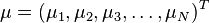## Just My Life & My Work### 非線性支持向量機器 (Non-linear SVMs)### 支持向量機器 (Support Vector Machine)（繼續閱讀…）

3D的時候不好看出結果，

test point有4個。

### [MatLab] 馬氏距離的理論與實做 (Mahalanobis Distance)

Mahalanobis distance

From WiKi (http://en.wikipedia.org/wiki/Mahalanobis_distance)

In statistics, Mahalanobis distance is a distance measure introduced by P. C. Mahalanobis in 1936. It is based on correlations between variables by which different patterns can be identified and analyzed. It is a useful way of determining similarity of an unknown sample set to a known one. It differs from Euclidean distance in that it takes into account the correlations of the data set and is scale-invariant. In other words, it is a multivariate effect size.

Definition

Formally, the Mahalanobis distance of a multivariate vectorfrom a group of values with meanand covariance matrix S is defined as:（繼續閱讀…）